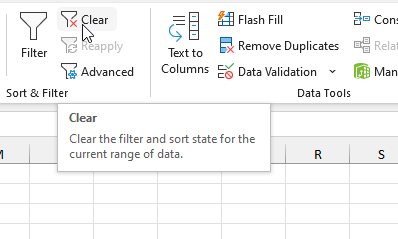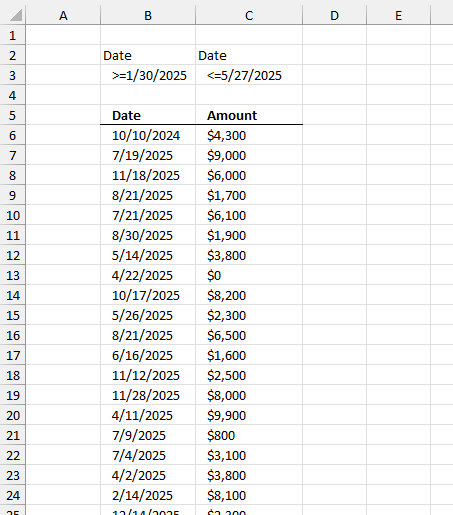Author: Oscar Cronquist Article last updated on January 17, 2023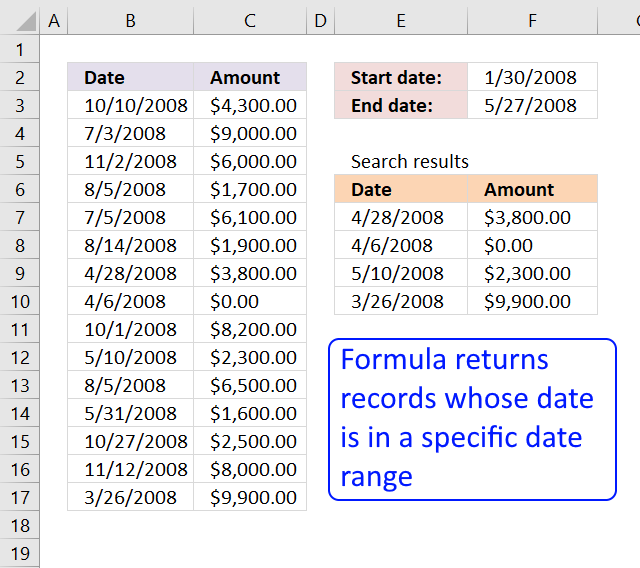This article presents methods for filtering rows in a dataset based on a start and end date.

The image above shows a formula in cells E7:F10 that extracts rows from cell range B3:C17 if the dates fall between the conditions specified in cells F2 and F3.

I will be showing you how to filter dates using these Excel features:

1. Excel 365 dynamic array formula - more powerful Excel functions.
2. Excel formula - earlier Excel versions.
3. Excel Table - easy to use.
4. Excel Table and VBA.
5. Autofilter - easy to use.
6. Advanced filter - handles more complicated criteria.

Dynamic array formulas require Excel 365, Excel Tables require Excel 2007 or a later version. The remaining methods should work on almost any Excel version.

## 1. Filter rows using array formulas based on a date range - Excel 365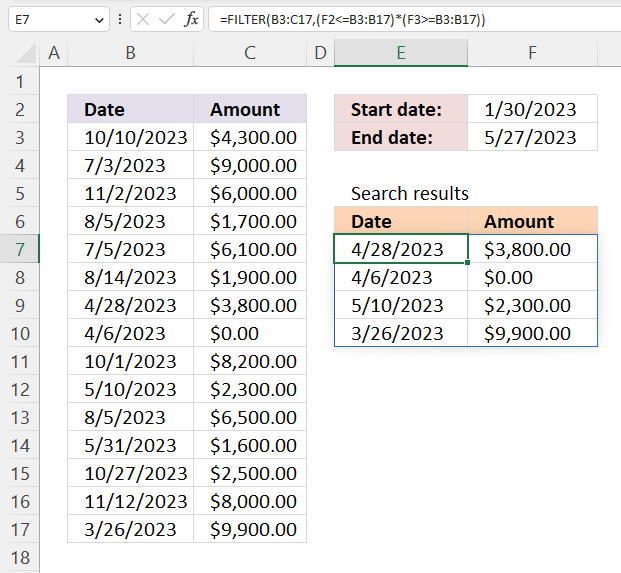This example shows the new FILTER function in cell E7, it uses the start and end dates to filter rows containing a date that falls between the start and end dates. The result is an array that is returned to cells below and to the right automatically.

Excel 365 dynamic array formula in cell E7:

=FILTER(B3:C17,(F2<=B3:B17)*(F3>=B3:B17))

Dynamic array formulas are a new feature in Excel 365 that allows you to enter a single formula in a cell and get results that automatically spill into other cells, as far as needed. This can save you time and make your formulas more efficient, as you don't need to create separate formulas for each cell or use the more complicated array formulas.

To create a dynamic array formula, you just need to enter the formula in a cell and press Enter. The results will automatically spill into the surrounding cells, as long as those cells are empty. You will get a a #SPILL error if adjacent cells are populated.

The dynamic array formula spills values to cells below or cells to the right depending on the result array size and shape.

### Explaining formula

The FILTER function lets you do amazing things that were not possible before, you needed much larger formulas in earlier Excel versions.

#### Step 1 - Compare dates to the start date

The less than, greater than, and equal signs are logical operators that let you compare values in Excel formulas, in this case, date values. The result is a logical value, in other words, a boolean value TRUE or FALSE.

Date values in Excel are actually stored as whole numbers. January 1, 1900, is the start date and each subsequent day is represented by a higher serial number. For example, January 1, 1900, is represented by the serial number 1, January 2, 1900, is represented by the serial number 2, and so on.

F2<=B3:B17

becomes

44956<={45209; 45110; 45232; 45143; 45112; 45152; 45044; 45022; 45200; 45056; 45143; 45077; 45226; 45242; 45011}

and returns

{TRUE; TRUE; TRUE; TRUE; TRUE; TRUE; TRUE; TRUE; TRUE; TRUE; TRUE; TRUE; TRUE; TRUE; TRUE}

#### Step 2 - Compare dates to the end date

F3>=B3:B17

becomes

45073>={45209; 45110; 45232; 45143; 45112; 45152; 45044; 45022; 45200; 45056; 45143; 45077; 45226; 45242; 45011}

and returns

{FALSE; FALSE; FALSE; FALSE; FALSE; FALSE; TRUE; TRUE; FALSE; TRUE; FALSE; FALSE; FALSE; FALSE; TRUE}

#### Step 3 - Multiply boolean values

Multiplying boolean values results in AND logic being performed, TRUE if all of the conditions are TRUE, and FALSE if any of the conditions are FALSE.

Here are the possible results of multiplying boolean values:

TRUE * TRUE = TRUE
TRUE * FALSE = FALSE
FALSE * TRUE = FALSE
FALSE * FALSE = FALSE

However, keep in mind Excel converts the result to their numerical equivalents:

TRUE - 1
FALSE - 0 (zero)

Also, in this particular example, Excel multiplies values that correspond to its position in the array meaning the first value in the first array is only multiplied by the first value in the second array and so on.

Using parentheses allows you to specify the order in which the operations should be performed. In this case, it is important to compare the values before multiplying them, as not doing so will result in incorrect output.

(F2<=B3:B17)*(F3>=B3:B17)

becomes

{TRUE; TRUE; TRUE; TRUE; TRUE; TRUE; TRUE; TRUE; TRUE; TRUE; TRUE; TRUE; TRUE; TRUE; TRUE}*{FALSE; FALSE; FALSE; FALSE; FALSE; FALSE; TRUE; TRUE; FALSE; TRUE; FALSE; FALSE; FALSE; FALSE; TRUE}

and returns

{0;0;0;0;0;0;1;1;0;1;0;0;0;0;1}

#### Step 4 - Filter rows based on logical test

The FILTER function extracts values/rows based on a condition or criteria.

Function syntax: FILTER(array, include, [if_empty])

FILTER(B3:C17,(F2<=B3:B17)*(F3>=B3:B17))

becomes

FILTER(B3:C17, {0;0;0;0;0;0;1;1;0;1;0;0;0;0;1})

and returns

{45044,3800; 45022,0; 45056,2300; 45011,9900}

## 2. Filter rows using array formulas based on a date range - earlier Excel versions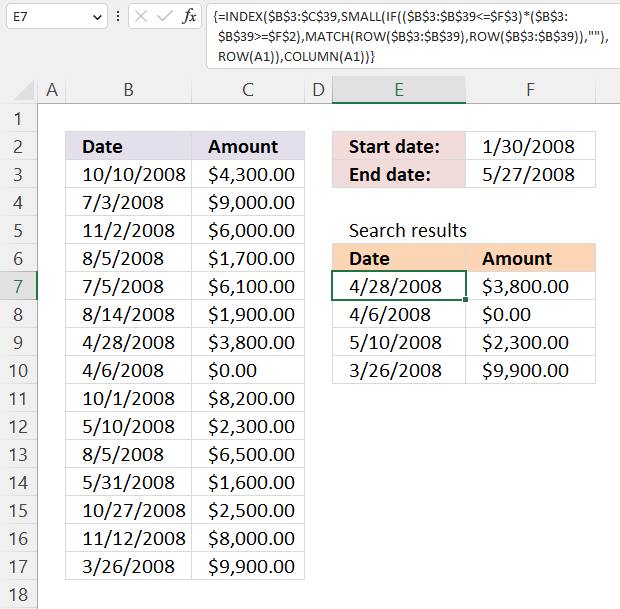Array formula in cell E7:

=INDEX(\$A\$2:\$B\$38, SMALL(IF((\$A\$2:\$A\$38<=\$H\$2)*(\$A\$2:\$A\$38>=\$H\$1), MATCH(ROW(\$A\$2:\$A\$38), ROW(\$A\$2:\$A\$38)), ""), ROW(A1)), COLUMN(A1))

### 2.1 How to create an array formula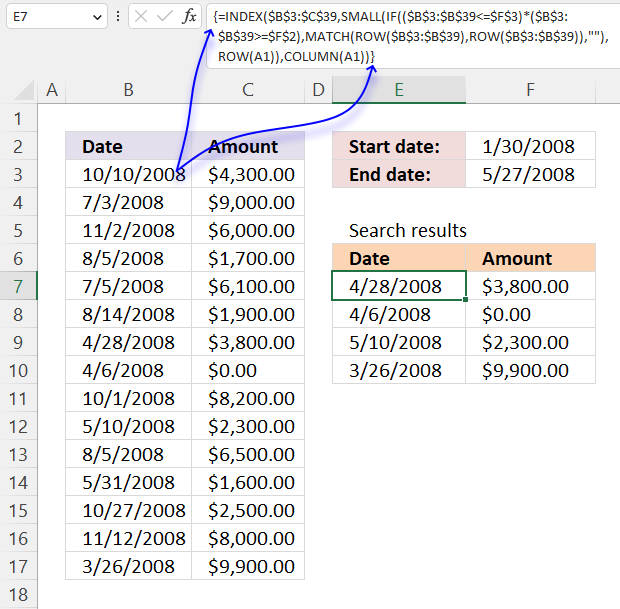1. Select cell E7.
2. Type the array formula.
3. Press and hold Ctrl + Shift simultaneously.
4. Press Enter once.
5. Release all keys.

The formula has a leading and trailing curly bracket like this:
{=INDEX(\$A\$2:\$B\$38, SMALL(IF((\$A\$2:\$A\$38<=\$H\$2)*(\$A\$2:\$A\$38>=\$H\$1), MATCH(ROW(\$A\$2:\$A\$38), ROW(\$A\$2:\$A\$38)), ""), ROW(A1)), COLUMN(A1))}

Don't enter these characters yourself, they appear automatically.

### 2.2 How to copy the array formula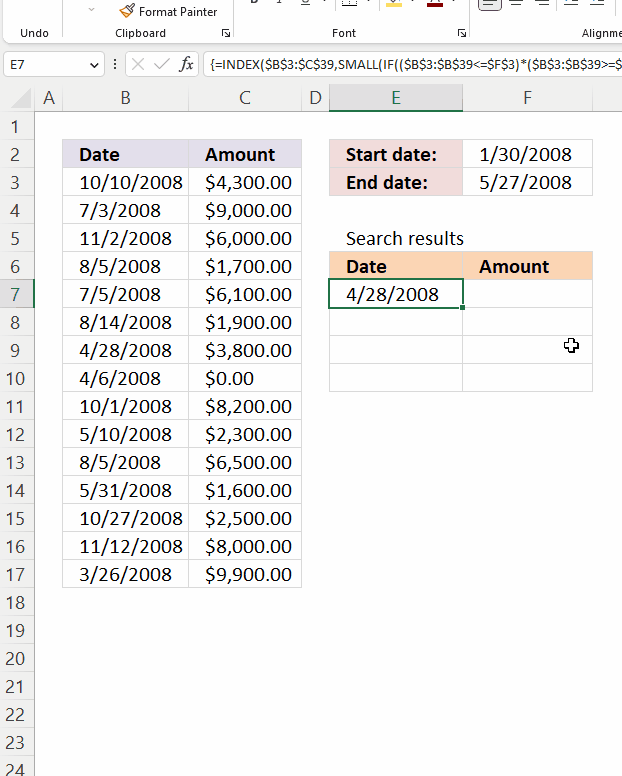1. Select cell E7.
2. Left-press and hold with the mouse button on the dot in the lower right corner of cell D7.
3. Drag to cell F7, release left mouse button.
4. Press the left mouse button and hold on the dot in the lower right corner of cell F7.
5. Drag down with the mouse as far as needed.
6. Release the left mouse button.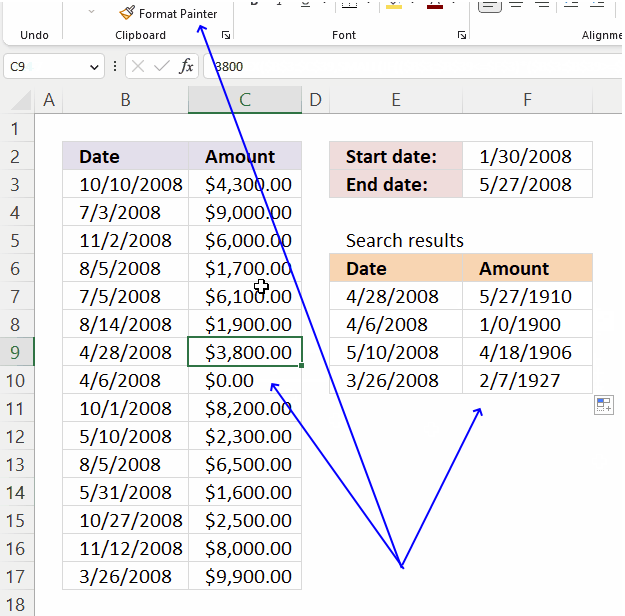How to change F7:F10 cell formatting:

1. Select a cell containing the source formatting, in this example any cell in C3:C17.
2. Double-press with left mouse button on the left mouse button on the "Format Painter" button on the ribbon tab "Home".
3. Select cell range F7:F10.

See the animated image above.### 2.3 How to remove #num errors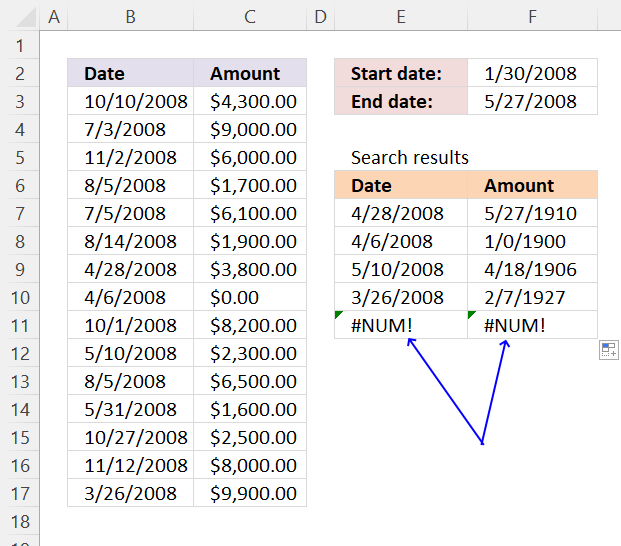=IFERROR(INDEX(\$A\$2:\$B\$38, SMALL(IF((\$A\$2:\$A\$38<=\$H\$2)*(\$A\$2:\$A\$38>=\$H\$1), MATCH(ROW(\$A\$2:\$A\$38), ROW(A2:\$A\$38)), ""), ROW(A1)), COLUMN(A1)),"")

The IFERROR function if the value argument returns an error, the value_if_error argument is used. If the value argument does NOT return an error, the IFERROR function returns the value argument.

Function syntax: IFERROR(value, value_if_error)

The IFERROR function handles all formula errors, this might be a problem for you. It makes it harder to spot and troubleshoot any other formula error than the #NUM error, why? You can't see the error on the worksheet.

### 2.4 Get Excel *.xls file

filter-records-between-two-dates.xls
(Excel 97-2003 Workbook *.xls)

## 3. Filter rows using an Excel tableThis example shows how to filter rows in an Excel Table based on a date range. First, we will convert the data set to an Excel Table. Second, we will learn how to filter the data using a start and end date.

### 3.1 Convert range to a table

1. Select range A2:B38
2. Go to tab "Insert"
3. Press with left mouse button on "Table"
4. Press with left mouse button on OK!

### 3.2 Filter dates

1. Press with left mouse button on Black arrow near Date header
2. Hover over "Date filters"
3. Press with left mouse button on "Between..."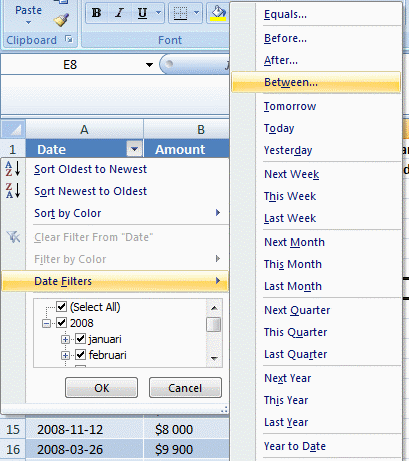4. Select dates5. Press with left mouse button on OK!

## 4. Filter rows in an Excel table using VBA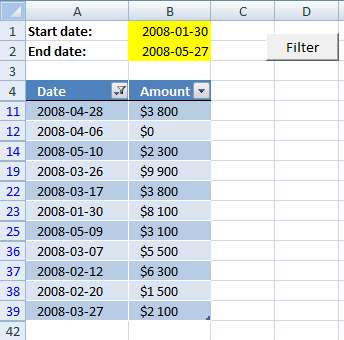In this example, you can type a date in cell B1 and B2. Press the button and the table is instantly filtered.

Copy the vba code below into a regular module. Create a button and assign the macro. There are more detailed instructions below.

### 4.1 VBA code

```Sub TblFilterDates()

Worksheets("Sheet3").ListObjects("Table13").Range.AutoFilter _
Field:=1, _
Criteria1:=">=" & Worksheets("Sheet3").Range("B1") _
, Operator:=xlAnd, _
Criteria2:="<=" & Worksheets("Sheet3").Range("B2")
End Sub
```

This VBA macro filters a table named "Table13" on a worksheet called "Sheet3" based on dates specified in cells B1 and B2, in the first column (Field:=1) of the table. The filter criteria are :

• Criteria1: ">=" & Worksheets("Sheet3").Range("B1")
This specifies that the dates in the first column of the table should be greater than or equal to the date in cell B1 on the "Sheet3" worksheet.
• Criteria2: "<=" & Worksheets("Sheet3").Range("B2")
This specifies that the dates in the first column of the table should be less than or equal to the date in cell B2 on the "Sheet3" worksheet.
• Operator:=xlAnd
This specifies that the filter should use "AND" logic to combine the two criteria meaning both criteria must match.

The AutoFilter method is used to apply a filter to a range of cells or a table in a worksheet. When a filter is applied, only the rows that meet the specified criteria are displayed, and the remaining rows are hidden. The AutoFilter method can be used to filter data based on a specific value, a range of values, or a pattern.

### 4.2 Where to copy vba code?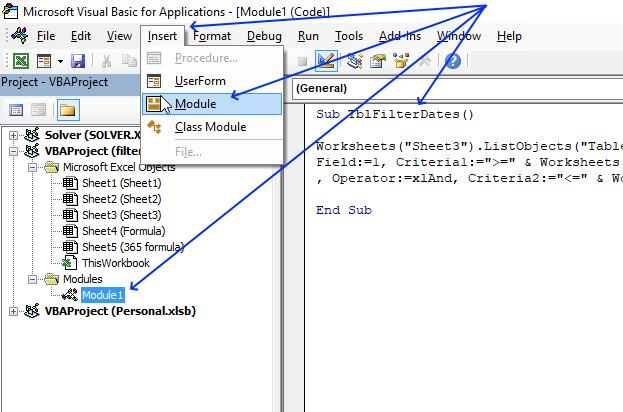1. Press Alt+F11 to open the Visual Basic Editor (VBE).
2. Press with mouse on Insert.
3. Press with mouse on "Module" to insert a new module to you workbook.
4. Copy and paste code into code window, see the image above.

### 4.3 How to create a button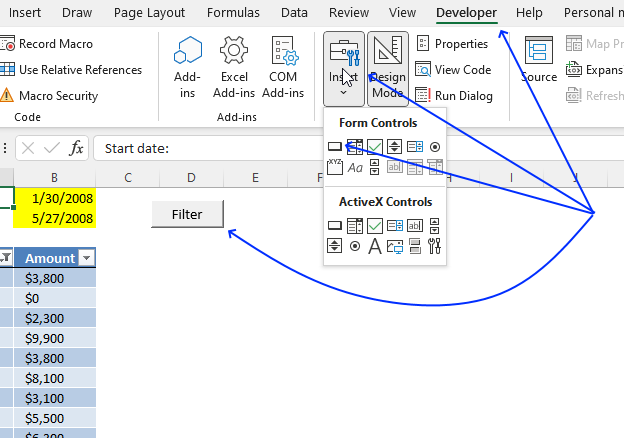1. Press with left mouse button on "Developer" tab on the ribbon.
2. Press with left mouse button on "Insert" button.
3. Create a button (form control) on the worksheet.
4. Press with right mouse button on on the button.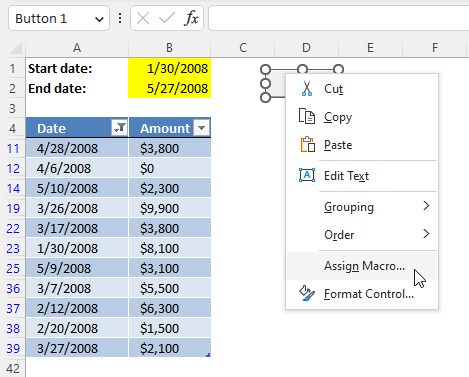A popup menu appears. Press with left mouse button on "Assign Macro...".
5. A dialog box shows up.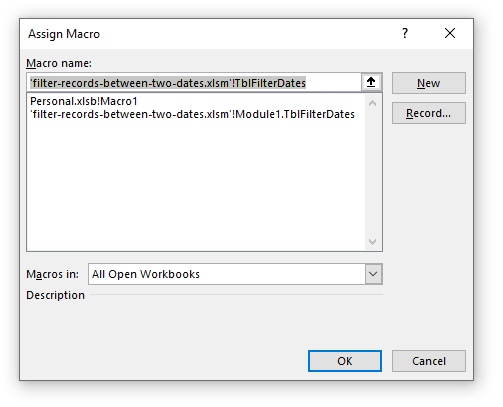6. Select the macro name.
7. Press with left mouse button on OK button.

### 4.4 Get Excel *.xlsm (macro-enabled) file

filter-records-between-two-dates.xlsm
(Excel 2007 MacroEnabled Workbook *.xlsm)

## 5. Filter rows based on a date range - Autofilter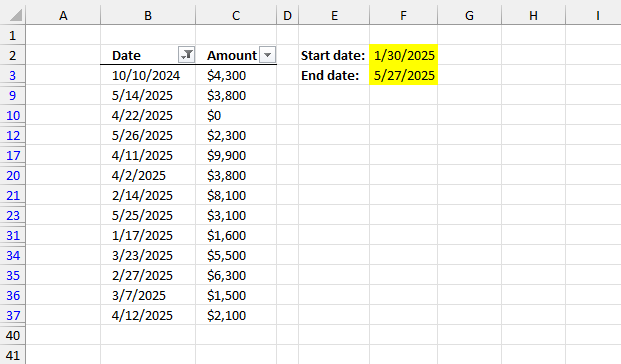This section demonstrates how to filter the data set in columns B and C using a start and end date.

### 5.1 Create Autofilter

1. Select any cell in the data set.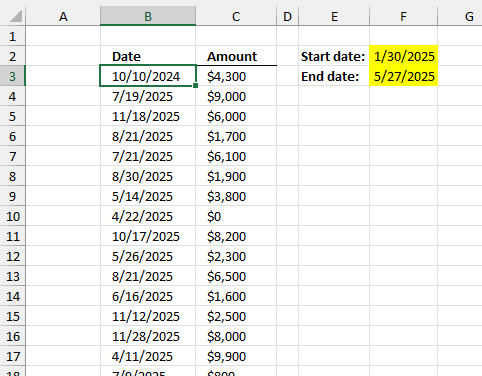2. Go to tab "Data" on the ribbon.
3. Press with left mouse button on the "Filter" button.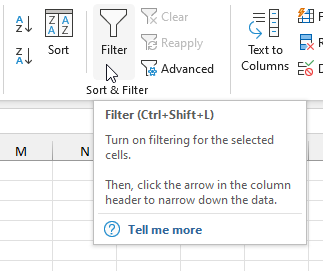4. The column headers now show a button containing an arrow.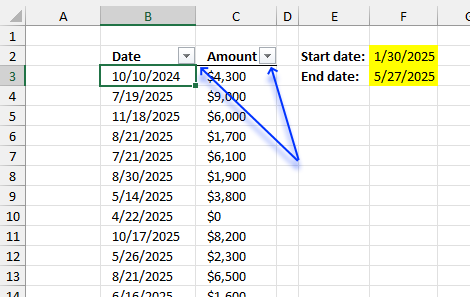### 5.2 Apply filter to data

1. Press with left mouse button on the arrow next to column header "Date". A popup menu appears.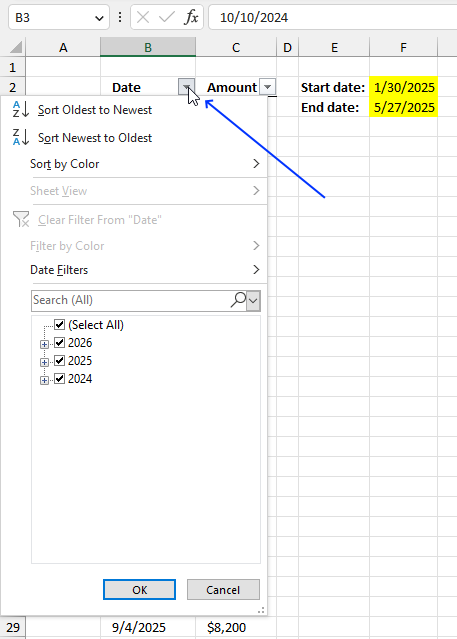2. Press with left mouse button on "Date Filters", and another menu appears.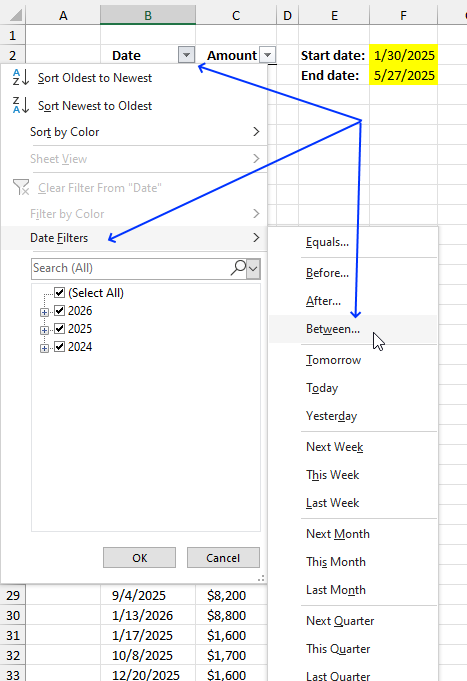3. Press with left mouse button on "Between...".
4. A dialog box appears, enter the start and end date.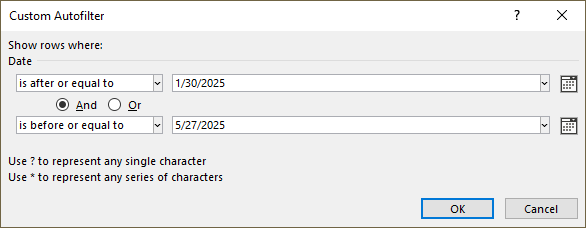5. Press with left mouse button on the "OK" button to apply changes.## 6. Filter rows based on a date range - Advanced filter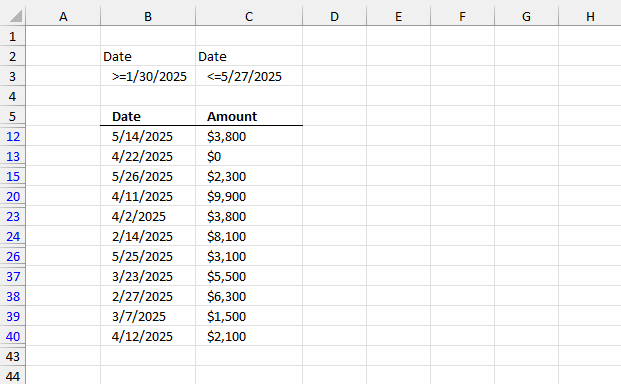This example demonstrates how to filter a data set based on a start and end date using "Advanced Filter".

### 6.1 Enter date range

1. Enter the column header name containing the dates in cells B2 and C2, they must match. My column header name is "Date" as shown in the image below.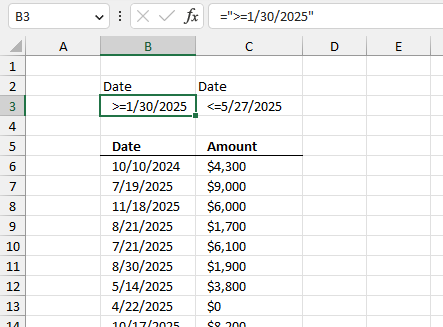2. Type =">=1/30/2025" in cell B3.
3. Press Enter.
4. Type ="<=5/27/2025" in cell C3.
5. Press Enter.

### 6.2 Start the "Advanced Filter"

1. Go to tab "Data" on the ribbon.
2. Press with mouse on the button named "Advanced".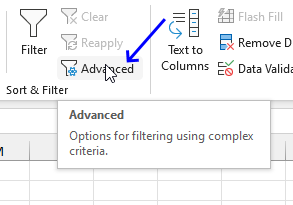3. A dialog box appears.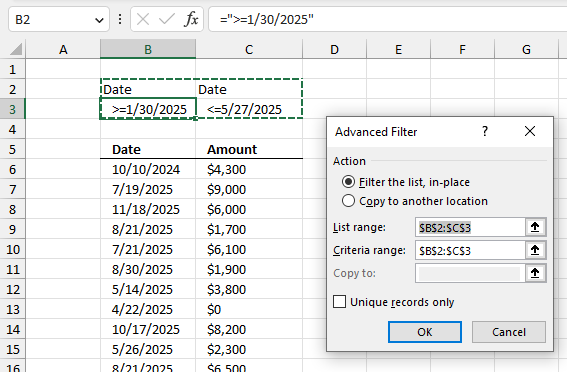4. Select the radio button named "Filter the list, in-place".
5. Press with left mouse button on the arrow in "List range:".
6. Select cell range B5:C42.
7. Press with left mouse button on the arrow in "Criteria range:".
8. Select cell range B2:C3.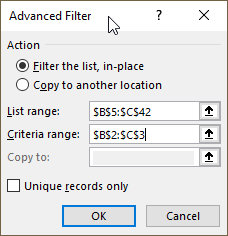9. Press with left mouse button on the "OK" button.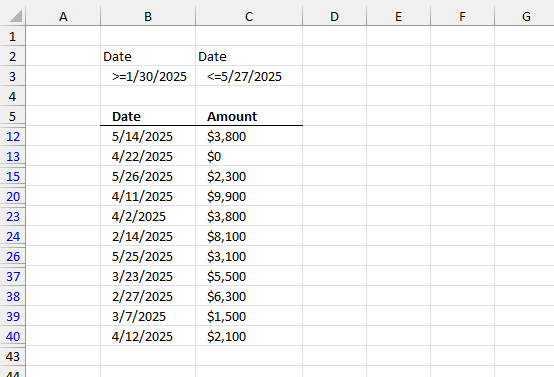The blue row numbers show that some sort of filter is applied, in this case, the "Advanced Filter".